# Tukey window

The Tukey window coefficients are given by the following formula

$$a(k)=\begin{cases} 0.5 (1+\cos(\frac{\pi(|k-M|-\alpha M)}{(1-\alpha)M})), \,\,\, |k-M| \ge \alpha M \\ 1, \,\,\, |k-M| \lt \alpha M \end{cases}$$

where N is the length of the filter, M = (N – 1) / 2, k = 0, 1, …, N – 1, and α is a constant between zero and one.

Consider a finite impulse response (FIR) low pass filter of length N = 201. The following is the Tukey window with α = 0.5.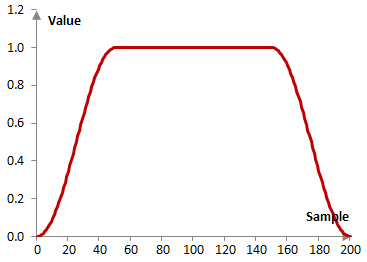Given a sampling frequency of 2000 Hz and a filter cutoff frequency of 40 Hz, the impulse response of the filter with a rectangular window (with no window) and with the Tukey window above is as follows.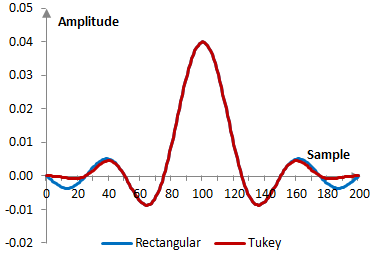The magnitude response of the same filter is shown on the graph below.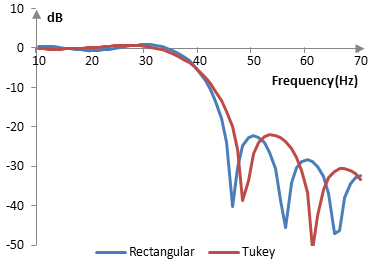A larger α implies a Tukey window with a "flatter" top, smaller transition band, and worse stop-band attenuation. As α approaches 1, the Tukey window itself approaches a rectangular window. A smaller α creates a Tukey window with a steeper top, larger transition band, and better stop-band attenuation. As α approaches 0, we have

$$a(k)=0.5 (1+\cos(\frac{2\pi|k-\frac{N-1}{2}|}{N-1}))=0.5 (1+\cos(\frac{2 \pi k}{N-1}-\pi))=0.5 (1+\cos(\frac{2 \pi k}{N-1}))$$

аnd the Tukey window approaches the Hann window.

The following is the Tukey window with three different values for α (0.3, 0.5, and 0.7).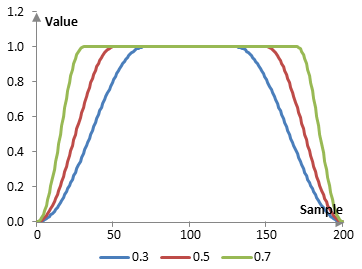The magnitude response of these same windows given the sampling frequency of 2000 Hz, cutoff frequency of 40 Hz, and a filter of length N = 201 is as follows.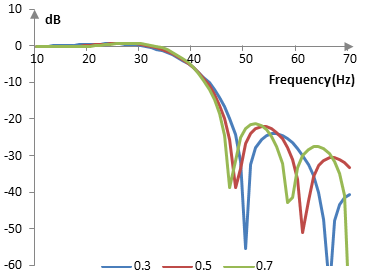## Measures for the Tukey window

The following is a comparison of the discrete Fourier transform of the Tukey window (α = 0.5) and the rectangular window.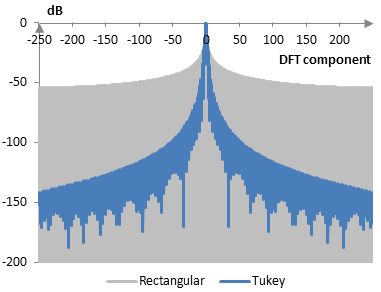The Tukey window measures are as follows.

 α 0.3 0.5 0.7 Coherent gain 0.65 0.75 0.85 Equivalent noise bandwidth 1.33 1.22 1.13 Processing gain -1.25 dB -0.88 dB -0.52 dB Scalloping loss -1.81 dB -2.23 dB -2.79 dB Worst case processing loss -3.06 dB -3.11 dB -3.31 dB Highest sidelobe level -18.2 dB -15.1 dB -13.8 dB Sidelobe falloff -16.3 dB / octave, -54.3 dB / decade -15.8 dB / octave, -52.6 dB / decade -15.3 dB / octave, -50.9 dB / decade Main lobe is -3 dB 1.28 bins 1.16 bins 1.04 bins Main lobe is -6 dB 1.76 bins 1.58 bins 1.42 bins Overlap correlation at 50% overlap 0.272 0.362 0.430 Amplitude flatness at 50% overlap 0.616 0.500 0.500 Overlap correlation at 75% overlap 0.710 0.727 0.738 Amplitude flatness at 75% overlap 0.978 1.000 0.776

Window

### This equation is the opposite

In that page, alpha is the proportion that is trigonometric.
I don't see a "proper definition," so nothing is wrong,
but it might be worth a comment to avoid confusing beginners.

### You are correct. In this

You are correct. In this definition, a larger alpha makes the window closer to a rectangular window. A smaller alpha makes it closer to a Hann window. In the Wikipedia definition, it is the opposite. Thanks.

### Filtered HTML

• Freelinking helps you easily create HTML links. Links take the form of [[indicator:target|Title]]. By default (no indicator): Click to view a local node.